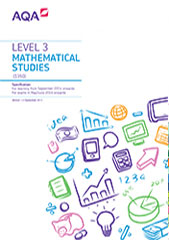# 3.3 Estimation

Students should become familiar with and gain confidence in ideas concerning the formulation of mathematical models.

## E1 The modelling cycle

Content

E1.1

representing a situation mathematically, making assumptions and simplifications

students will engage in the tackling of 'open' mathematical problem-solving where there may not be a clear single approach or 'correct' answer

E1.2

selecting and using appropriate mathematical techniques for problems and situations

E1.3

interpreting results in the context of a given problem

E1.4

evaluating methods and solutions including how they may have been affected by assumptions made

## E2 Fermi estimation

Content